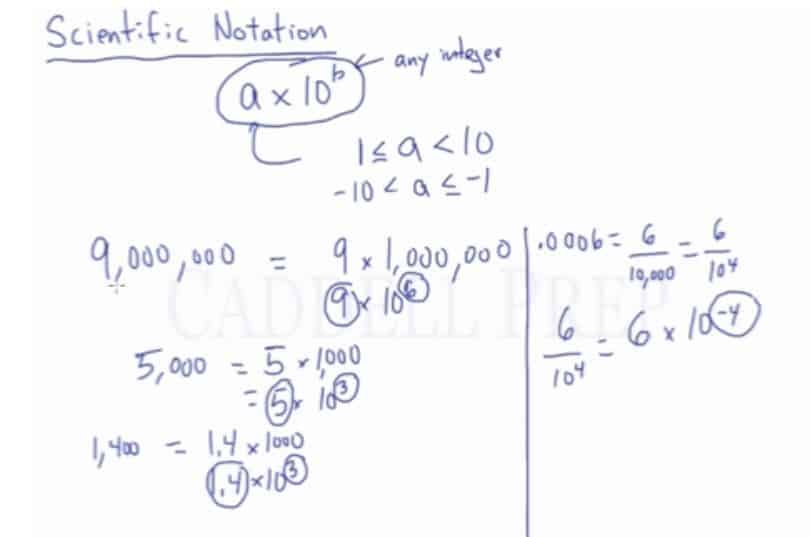## Example 1$0.000016$

First, let’s get a number between$1$ and$10$

That is$1.6$

Then, let’s count the decimal spaces to have the value of our exponent

We counted$5$ decimal spaces

So we’ll have$0.000016= 1.6 \times 10^5$

Since, we counted from left to right. We have to counteract that by making the value of our exponent negative$0.000016= 1.6 \times 10^{-5}$

## Example 2$23,400,000$

First, let’s get a number between$1$ and$10$

That is$2.34$

Then, let’s count how many decimal spaces are there in$23,400,000$ for us to get$2.34$

There are$7$ decimal spaces.

Since, we counted from the right to left. Meaning it’s a big number and we’re making it small.

Now, we have:$23,400,000= 2.34\times 10^7$

## Video-Lesson Transcript

In this lesson, you will learn how to write numbers in scientific notation. After you finish this lesson, view all of our Pre-Algebra lessons and practice problems.

The basic form of scientific notation is$a \times 10^b$

Whereas$b$ can be any integer and can be positive or negative.

Whereas$a$ is$1 \leq a \textless 10$

Or$a$ can also be negative$-10 \textless a \leq -1$

Whereas$10$ is constant.

Here’s an example:$9,000,000 = 9 \times 1,000,000$

It’s really$= 9 \times 10^6$

Let’s say we have$5,000$

It’s really$= 5 \times 1,000$

and then$= 5 \times 10^3$

If we have$1,400$

it could be written as$= 1.4 \times 1,000$

which really is$= 1.4 \times 10^3$

See$9$,$5$, and$1.4$ are our$a$. All of them meet the requirement above.

Likewise, all the exponents are integers.

Here’s an example of a negative exponent.$0.0006$

This is$= \dfrac{6}{10,000}$

which is$= \dfrac{6}{(10^4)}$

To review our lesson in Exponents, we have to change the sign to move the denominator to the numerator.

So since we want to move the denominator to the numerator, we have to write$= 6 \times 10^{-4}$This is a bit tedious work.

Don’t worry. There’s a quicker way to do this!

## Writing Scientific Notation by Counting Decimal Places

For example, we have$3,000$

Let’s get$a$ where$1 \leq a \textless 10$ then multiplied by$10$

So it’s$= 3 \times 10$

Then let’s count the decimal spaces from the right of$3,000$ and stop to get$3$.

We counted$3$ decimal spaces.

This goes right in as our exponent.

So now we have$3,000 = 3 \times 10^3$

Let’s have another one.$54,000$

Remember, we need to have a number that is less than$10$ so we can’t have$54$.

Instead, we can have$5.4$

So, we’ll have$5.4 \times 10$

Then let’s count how many decimal spaces are there in$54,000$ for us to get$5.4$

There are$4$ decimal spaces. This goes right in as our exponent.

We counted from the right to left. Meaning it’s a big number and we’re making it small.

Now, we have$54,000 = 5.4 \times 10^4$

Now, let’s have a small number.$0.0002$

Let’s get a number between$1$ and$10$.

That is$2$

Multiplied by$10$ which is constant

Then let’s count the decimal spaces to have the value of our exponent.

We counted$4$ decimal spaces.

So we’ll have$0.0002 = 2 \times 10^4$

But remember we counted from left to right. Which means it’s a small number and we’re making it big number.

We have to counteract that by making the value of our exponent negative.

So our final answer is$0.0002 = 2 \times 10^{-4}$

Exponent should be in negative if we have a small number and we’re writing it to appear a big number.

On the other hand, an exponent is positive if our original number is big and we’re writing it to become a small number.

Let’s have one more example.

We have$0.0056$

The number between$1$ and$10$ is$5.6$

Our exponent is$3$ since we moved three decimal spaces to get$5.6$.

Moreover, it should be in negative because we have a small number.

So the scientific notation is$0.0056 = 5.6 \times 10^{-3}$

Another way to look at the value of exponent:

If you moved the decimal to the left, you’ll have a positive exponent.

And if you moved the decimal to the right, you’ll have a negative exponent.